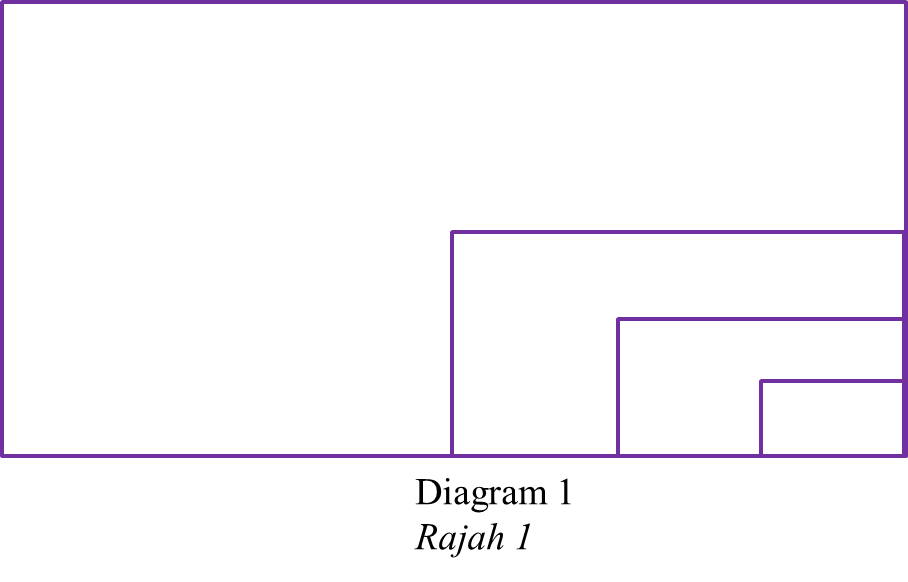# SPM Additional Mathematics (Model Test Paper) – Q&A 3-4

SPM Additional Mathematics (Model Test Paper)

Section A
[40 marks]

Question 3
Diagram 1 shows four rectangles. The largest rectangle has a length of L cm and a width of W cm. The measurement of the length and width of each subsequent rectangle are half of the measurements of its previous one. The areas of the rectangles form a geometric progression. The terms of the progression are in descending.(a) State the common ratio, hence find the area of the first rectangle given the sum of four rectangles is 510 cm2. [4 marks]
(b) Determine which rectangle has an area of 96 cm2.  [2 marks]

(a)
$\begin{array}{l}{A}_{1}=LW\\ {A}_{2}=\left(\frac{1}{2}L\right)\left(\frac{1}{2}W\right)=\frac{LW}{4}\\ {A}_{3}=\left(\frac{1}{4}L\right)\left(\frac{1}{4}W\right)=\frac{LW}{16}\\ \text{Common ration,}r=\frac{\frac{LW}{4}}{LW}=\frac{1}{4}\end{array}$

$\begin{array}{l}{S}_{n}=\frac{a\left(1-{r}^{n}\right)}{1-r}\\ {S}_{4}=510\\ \frac{a\left(1-{r}^{4}\right)}{1-r}=510\\ \frac{a\left[1-{\left(\frac{1}{4}\right)}^{4}\right]}{1-\frac{1}{4}}=510\\ \frac{255a}{256}=\frac{765}{2}\\ a=\frac{765}{2}×\frac{256}{255}\\ a=384\end{array}$

Area of the first rectangle = 384 cm2

(b)
$\begin{array}{l}{T}_{n}=96\\ a{r}^{n-1}=96\\ 384{\left(\frac{1}{4}\right)}^{n-1}=96\\ {\left(\frac{1}{4}\right)}^{n-1}=\frac{96}{384}\\ {\left(\frac{1}{4}\right)}^{n-1}={\left(\frac{1}{4}\right)}^{1}\\ n-1=1\\ n=2\end{array}$

The second rectangle has an area of 96 cm2.

Question 4
(a) A worker is pumping air into a spherical shape balloon at the rate of  25 cm3 s-1.
$\left[\text{Volume of a sphere},\text{}V=\frac{4}{3}\pi {r}^{3}\right]$
Leaving answer in terms of π, find,
(i) rate of change of radius of the balloon when its radius is 10 cm.   [3 marks]
(ii) approximate change of volume when radius of the balloon decrease from 10 cm to  9.95 cm.    [2 marks]
(b) A curve $y=h{x}^{3}-\frac{3}{x}$  has turning point x = 1, find value of h.  [3 marks]

$\begin{array}{l}V=\frac{4}{3}\pi {r}^{3}\\ \frac{dV}{dr}=4\pi {r}^{2}\\ \frac{dr}{dt}=\frac{dr}{dV}×\frac{dV}{dt}\\ \text{}=\frac{1}{4\pi {r}^{2}}×25\\ \text{}=\frac{1}{4\pi {\left(10\right)}^{2}}×25\\ \text{}=\frac{1}{16\pi }\end{array}$
$\begin{array}{l}\frac{\delta V}{\delta r}\approx \frac{dV}{dr}\\ \delta V=\frac{dV}{dr}×\delta r\\ \text{}=4\pi {r}^{2}×\left(9.95-10\right)\\ \text{}=4\pi {\left(10\right)}^{2}×\left(-0.05\right)\\ \text{}=-20\pi \end{array}$